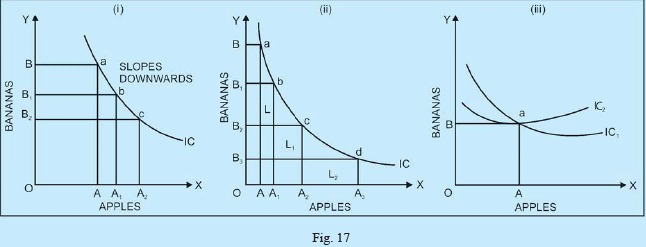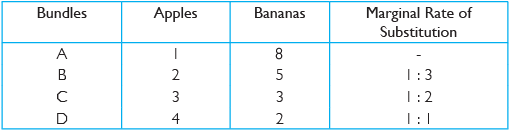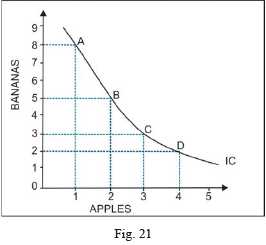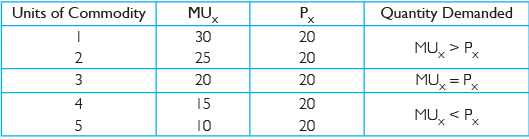Courses

# Long Questions With Answers - Theory Of Consumer Behaviour Commerce Notes | EduRev

## Commerce : Long Questions With Answers - Theory Of Consumer Behaviour Commerce Notes | EduRev

The document Long Questions With Answers - Theory Of Consumer Behaviour Commerce Notes | EduRev is a part of the Commerce Course Economics Class 11.
All you need of Commerce at this link: Commerce

Q.1. Explain the three properties of indifference curves.
Ans.
Following are the properties of indifference curves.
(i) Indifference Curve Slopes Downwards From Left to Right: An important property of an indifference curve is that it has a negative slope, that is, it slopes downwards from left to right. An indifference curve slopes downward because more of one good means less of the other if Total Utility is to remain unchanged. Refer part (i) of the diagram.
(ii) Indifference Curve is Convex to the Origin: An indifference curve is convex to the origin. Its slope diminishes or becomes flatter as we move downwards, This property of indifference curve follows from the fact that Marginal Rate of Substitution of apples for bananas diminishes as more and more of apples are substituted for bananas, Refer part (ii) of the diagram.
(iii) Two Indifference Curves Never Intersect: Another important property of indifference curve analysis is that two indifference curves cannot intersect each other. The two indifference curves, IC1, and IC2, represent two different levels of satisfaction. If these indifference curves intersect each other, the point of intersection will represent same level of satisfaction, which is not possible. Refer part (iii) of the diagram.Q.2. Explain the concept of Marginal Rate of Substitution with the help of a numerical example. Also explain its behaviour along an indifference curve.
Ans.
The Marginal Rate of Substitution (MRS) measures the rate at which the consumer is just willing to substitute one good for the other, maintaining the same level of satisfaction. It is the slope of the indifference curve.
When a consumer gets an additional unit of one good and gives up some units of the other goods, his or her satisfaction remains the same. In this case, the utility gained is equal to the utility lost. As the amount consumed of good I increases, the Marginal Rate of Substitution between good I and good 2 diminishes. This is the law of Diminishing Marginal Rate of Substitution.
According to Prof. Bilas, "The Marginal Rate of Substitution of X for Y (MRSXY) is defined as the amount of Y, the consumer is just willing to give up to get one more unit of X and maintains the same level of satisfaction."
The Marginal Rate of Substitution can be explained with the help of the following schedule:The schedule indicates that the consumer yjves up 3 banns for getting the 2nd apple, 2 bananas for getting the 3rd apple and I banana for getting the 4th apple. This shows that the Marginal Rate of Substitution along an indifference curve goes on diminishing as more and more apples are substituted for bananas. The diagram represents the indifference curve (IC) for the above schedule. As the consumer moves downwards, from point A to point B, he or she is willing to give up 3 bananas to obtain I apple. As the consumer moves further to the right, his or her willingness to give up bananas for an additional apple declines. The slope of the indifference curve declines as it becomes flatter towards the right. This is the Law of Diminishing Marginal rate of Substitution.Q.3. Explain consumer's equilibrium in case of a single commodity with the help of a utility schedule.
Ans.
The single commodity equilibrium condition states that a consumer is in equilibrium and derives maximum satisfaction when Marginal Utility of a commodity is equal to its price. This condition of consumer equilibrium can be represented with the help of the following equation:
MUx = Px

(i) When MUx > Px
When the Marginal Utility of commodity X is more than its price (MUx > Px) then an increased purchase of the commodity X will increase consumer's Marginal Utility. That is, the satisfaction and welfare of the consumer will increase. The consumer will continue to buy more units of the commodity and the Marginal Utility derived from additional unit will fall until MU becomes equal to price again.
(ii) When MUx < Px
When the Marginal Utility of commodity X is less than its price (MUx < Px) then a reduced purchase of the commodity X will decrease consumer's Marginal Utility. That is, the satisfaction and welfare of the consumer will decrease. The consumer will continue to reduce the units of the commodity and the Marginal Utility derived from additional unit will increase until MU becomes equal to price again.
Description of the equilibrium of consumer can be shown with the help of the following schedule:It is clear from the schedule that consumer will be in equilibrium when he or she is purchasing three units because at this point MUx is equal to price Px. The consumer is getting maximum satisfaction. Thus, he or she will not want to change the condition of equilibrium. Any deviation from this point will lead to a fall in consumer's satisfaction.

,

,

,

,

,

,

,

,

,

,

,

,

,

,

,

,

,

,

,

,

,

;